# Interpolation formula

(diff) ← Older revision | Latest revision (diff) | Newer revision → (diff)

A formula for the approximate calculation of values of a functionby replacing it by a functionthat is simple in a certain sense and belongs to a certain class. The parameters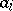,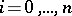, are chosen in such a way that the values of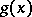coincide with the known values ofon a given set ofdistinct values of the argument:(1)

This method of approximately representing a function is called interpolation, and the points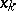at which (1) should hold are called interpolation nodes. Instead of the simplest condition (1), the values of some quantity related tomay also be given, e.g. the values of a derivative ofat interpolation nodes.

The method of linear interpolation is the most widespread among the interpolation methods. The approximation is now looked for in the class of (generalized) polynomials(2)

in some fixed system of functions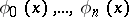. In order for the interpolation polynomial (2) to exist for any functiondefined on an interval, and for any choice ofnodes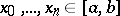,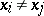if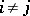, it is necessary and sufficient thatis a Chebyshev system of functions on. The interpolation polynomial will, moreover, be unique and its coefficientscan be found by directly solving (1).

Forone often takes: the sequences of powers of,the sequence of trigonometric functions,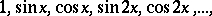or the sequence of exponential functions,where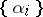is a sequence of distinct real numbers.

When interpolating by algebraic polynomials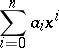(3)

the system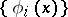is(4)

while (1) has the form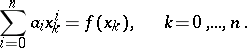(5)

The system (4) is a Chebyshev system, which ensures the existence and uniqueness of the interpolation polynomial (3). A property of (4) is the possibility of obtaining an explicit representation of the interpolation polynomial (3) without immediately having to solve (5). One of the explicit forms of (3),(6)

is called the Lagrange interpolation polynomial (cf. Lagrange interpolation formula). If the derivative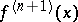is continuous, the remainder of (6) can be written as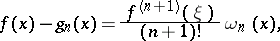(7)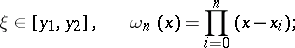where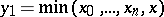;. The value of the remainder (7) depends, in particular, on the values of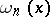. The choice of interpolation nodes for whichis minimal, is of interest. The distribution of the nodes is optimal in this sense if the rootsof the polynomialwhich deviates least from zero on, are taken as the nodes. Here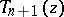is the Chebyshev polynomial of degree(cf. Chebyshev polynomials).

There is a number of other explicit representations of (3) that are more useful for solving this or another practical interpolation problem (cf., e.g., Bessel interpolation formula; Gauss interpolation formula; Newton interpolation formula; Stirling interpolation formula; Steffensen interpolation formula; Everett interpolation formula). If it is difficult to estimate in advance the degree of the interpolation polynomial that is necessary for attaining the error desired (e.g., when interpolating a table), then one takes recourse to the Aitken scheme. In this scheme interpolation polynomials of increasing degrees are constructed sequentially, thus making it possible to control the accuracy in the computational process. Another approach to the construction of interpolation formulas can be found in Fraser diagram.

The Hermite interpolation formula gives the solution to the problem of the algebraic interpolation of the values of a function and its derivatives at interpolation nodes.

How to Cite This Entry:
Interpolation formula. Encyclopedia of Mathematics. URL: http://encyclopediaofmath.org/index.php?title=Interpolation_formula&oldid=14575
This article was adapted from an original article by M.K. Samarin (originator), which appeared in Encyclopedia of Mathematics - ISBN 1402006098. See original article